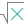#COMBINA (PQL - xl)

This function calculates the number of possible combinations (along with repetitions) for the specified number of items

• Library: PQL \ Spreadsheet \ Math & Trig
• Compatibility: Any content (regardless of data source) in the Tabulate spreadsheet module
• Solve: This function can be used with the Solve plug-in: both as part of the objective function and constraint functions

#### Syntax

COMBINA(number, number chosen)

##### Function Arguments
 Name Description Type Optional number Refers to the number representing the number of items. This value must be positive and greater than or equal to the second argument i.e. number chosen Number number chosen Refers to the number of items in each possible combination. This value must be positive Number